# Quiz 15: Risk-Pooling Strategies to Reduce and Hedge Uncertainty

Business

The variances of two or more independent random variables can be added up to get the overall variance. So, if there are n number of independent demand centres, the variance of a replacement consolidated centre will be the sum of variances of these independent centres. The standard deviation of the consolidated centre can off-course be found by taking the square root of its variance. a. Note that the standard deviation of the weekly demand in each of the single warehouses is 30. So, the variance is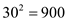.So, the standard deviation of the consolidated warehouse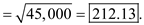b. Compute the pipeline inventory as follows:So, there will be a pipeline inventory of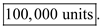The coefficient of variation (CV) of two independent random variables can be computed by knowing their individual coefficient of variation and the correlation between them. The following formula can be used.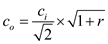Where,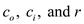are the total CV, individual CV, and correlation coefficient respectively. Note the given information: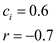Compute the coefficient of variation for total demand as follows: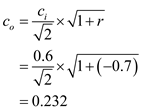So, the coefficient of variation of total demand is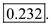In a base stocking policy, the ordering is done is a fixed interval. The order-up-to level is decided based on the average demand during the lead time plus review period, its standard deviation, and the required service level percentage. The expected ending inventory at the end of the period can be computed using the following formula: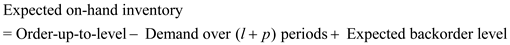Where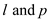stand for the replenishment lead time and review period respectively. a. Note the given data: Average weekly demand, d = 1.25 Review period, p = 1 week Delivery lead time,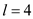days Compute the total average ending inventory using the following method: The in-stock probability is 0.95. From the Poisson table, F (11) = 0.975 and F (10) = 0.946. So, the appropriate order-up-to level,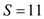Form the Poisson distribution table, for L (11) = 0.0467. This is the expected backorder level.For 200 SKUs, the total inventory level is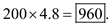b. Note the given data: Average weekly demand, d = 50 Standard deviation of weekly demand,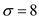Review period, p = 1 week Delivery lead time,days Compute the total average ending inventory using the following method: The required in-stock probability is 95%. The corresponding value of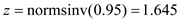The corresponding value of loss function is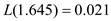So, the expected back-order level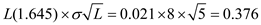For 5 SKUs, the total inventory level is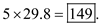c. Compare the inventory holding cost before and after the improvement in fill rate as follows: For the in-stock probability of 98%, the corresponding value of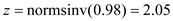The corresponding value of loss function is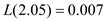So, the expected back-order level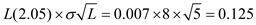For 5 SKUs, the total inventory level is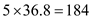The holding cost becomes equal to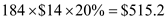The holding cost with initial condition was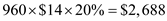So, the change in store's holding cost to the original situation is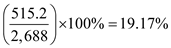. So, the change in store's holding cost to the original situation is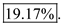There is no answer for this question